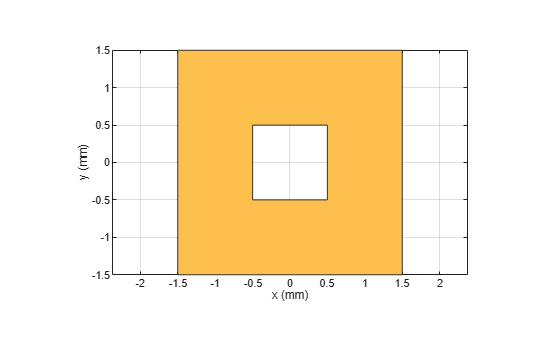# and

Shape1 & Shape2 for RF PCB shapes

Since R2021b

## Syntax

``c = and(shape1,shape2)``

## Description

example

````c = and(shape1,shape2)` calls the syntax `shape1 & shape2` to intersect two shapes.```

## Examples

collapse all

Create and display an annular ring.

```shape1 = ringAnnular(InnerRadius=1e-3); show(shape1)```Create and display a square ring.

```shape2 = ringSquare(InnerSide=1e-3); show(shape2)```Find and display the intersection of the shapes.

```shapeIntersection = and(shape1,shape2); show(shapeIntersection)```Create and display an annular ring.

`shape1 = ringAnnular;`

Create and display a square ring.

`shape2 = ringSquare('InnerSide',8e-3);`

Find and display the intersection of the shapes.

```shapeIntersection = shape1&shape2; show(shapeIntersection)```## Input Arguments

collapse all

First shape created using custom elements and shape objects of RF PCB Toolbox™, specified as an object.

Example: `shape1 = bendCurved;` specifies the first shape as a `bendCurved` object.

Second shape created using custom elements and shape objects of RF PCB Toolbox, specified as an object.

Example: `shape2 = ringAnnular;` specifies the second shape as a `ringAnnular` object.

## Version History

Introduced in R2021b